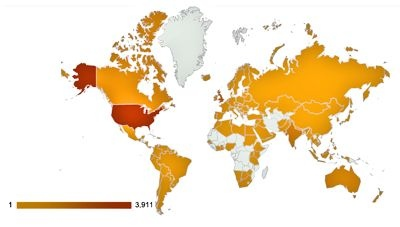# Global ReadershipI quite like WordPress.com’s latest data visualisation tool, which allows you to see the spread of people reading your blog. The data only goes back to February 25th 2012 and presumably a number of hits can have no country attributed to them, but it’s still a nice addition and interesting for me to see the number of different places that readers come from.

Perhaps we’ll gloss over the Mercator Projection and also how annoying and fiddly the WordPress app for iOS is; then things are always harder on iPad – right?

# The triangle paradox – solved

When I posted The triangle paradox, I said that I would post a solution in few days. As per the comments on my earlier article, some via Twitter and indeed the context of the article in which this supposed mathematical conundrum was posted, the heart of the matter is an optical illusion.

If we consider just the first part of the paradox:Then the key is in realising that the red and green triangles are not similar (in the geometric sense of the word). In particular the left hand angles are not the same, thus when lined-up they do not form the hypotenuse of the larger, compound triangle that our eyes see. In the example above, the line tracing the red and green triangles dips below what would be the hypotenuse of the big triangle. In the rearranged version, it bulges above. This is where the extra white square comes from.

It is probably easier to see this diagrammatically. The following figure has been distorted to make things easier to understand:Let’s start with my point about the triangles not being similar:EAB = tan-1(2/5) ≈ 21.8°FAC = tan-1(3/8) ≈ 20.6°

So the two triangles are not similar and, as stated above, the two arrangements don’t quite line up to form the big triangle shown in the paradox. There is a “gap” between them formed by the grey parallelogram above, whose size has been exaggerated. This difference gets lost in the thickness of the lines and also our eyes just assume that the two arrangements form the same big triangle.

To work out the area of the parallelogram:

AE = (22 + 52)½ = √29
EI = (32 + 82)½ = √73
AI = (52 + 132)½ = √194

The area of a triangle with sides a, b and c is given by:Sparing you the arithmetic, when you substritute the values for AE, EI and AI in the above equation, the area of ∆ AEI is precisely ½.

∆ AEI and ∆ AFI are clearly identical, so the area of parallelogram AEIF is twice the area of either is

2 x ½ = 1

This is where the “missing” square comes from.

As was pointed out in a comment on the original post, the above should form something of a warning to those who place wholly uncritical faith in data visualisation. Much like statistics, while this is a powerful tool in the hands of the expert, it can mislead if used without due care and attention.